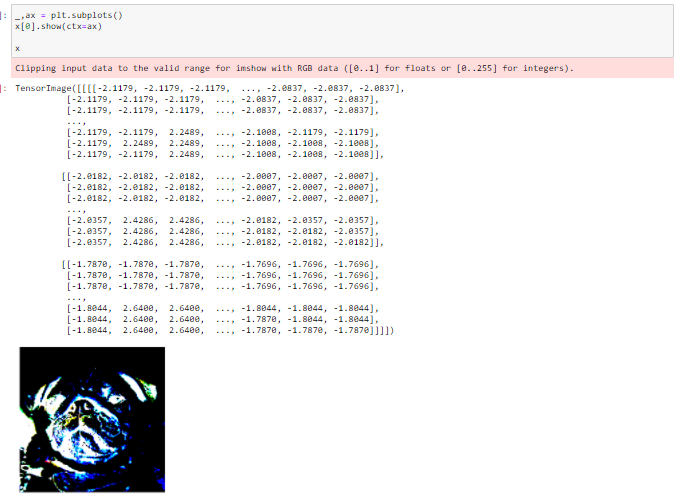# Default color space transformations

Hi, I was following the CAM chapter of fastai2 tutorials.

``````path = untar_data(URLs.PETS)/'images'
def is_cat(x): return x.isupper()
path, get_image_files(path), valid_pct=0.2, seed=21,
label_func=is_cat, item_tfms=Resize(224))
learn = cnn_learner(dls, resnet34, metrics=error_rate)
learn.fine_tune(1)

class Hook():
def hook_func(self, m, i, o): self.stored = o.detach().clone()
hook_output = Hook()
hook = learn.model.register_forward_hook(hook_output.hook_func)

img = dls.train.one_batch()
x, = first(dls.test_dl([img]))

output = learn.model.eval()(x)
F.softmax(output, dim=-1)

hook_output.stored.shape

act = hook_output.stored
cam_map = torch.einsum('ck,kij->cij', learn.model[-1].weight, act)

x_dec = TensorImage(dls.train.decode((x,2)))
_,ax = plt.subplots()
x_dec.show(ctx=ax)
x_dec
``````

I am very confused about the color space here. So I also plotted x and img:I know I loaded the picture from dls. How should I properly display the photo tho? Would greatly appreciate your help. This isn’t the first time that this issue has occurred.